$$\newcommand{\vecs}{\overset { \rightharpoonup} {\mathbf{#1}} }$$ $$\newcommand{\vecd}{\overset{-\!-\!\rightharpoonup}{\vphantom{a}\smash {#1}}}$$$$\newcommand{\id}{\mathrm{id}}$$ $$\newcommand{\Span}{\mathrm{span}}$$ $$\newcommand{\kernel}{\mathrm{null}\,}$$ $$\newcommand{\range}{\mathrm{range}\,}$$ $$\newcommand{\RealPart}{\mathrm{Re}}$$ $$\newcommand{\ImaginaryPart}{\mathrm{Im}}$$ $$\newcommand{\Argument}{\mathrm{Arg}}$$ $$\newcommand{\norm}{\| #1 \|}$$ $$\newcommand{\inner}{\langle #1, #2 \rangle}$$ $$\newcommand{\Span}{\mathrm{span}}$$ $$\newcommand{\id}{\mathrm{id}}$$ $$\newcommand{\Span}{\mathrm{span}}$$ $$\newcommand{\kernel}{\mathrm{null}\,}$$ $$\newcommand{\range}{\mathrm{range}\,}$$ $$\newcommand{\RealPart}{\mathrm{Re}}$$ $$\newcommand{\ImaginaryPart}{\mathrm{Im}}$$ $$\newcommand{\Argument}{\mathrm{Arg}}$$ $$\newcommand{\norm}{\| #1 \|}$$ $$\newcommand{\inner}{\langle #1, #2 \rangle}$$ $$\newcommand{\Span}{\mathrm{span}}$$$$\newcommand{\AA}{\unicode[.8,0]{x212B}}$$

The construction of linear combinations of the basis of atomic movements allows the vibrations belonging to irreducible representations to be investigated. The wavefunction of these symmetry equivalent orbitals is referred to as Symmetry Adapted Linear Combinations, or SALCs. SALCs (Symmetry Adapted Linear Combinations) are the linear combinations of basis sets composed of the stretching vectors of the molecule. The SALCs of a molecule can help determine binding schemes and symmetries. The procedure used to determine the SALCs of a molecule is also used to determine the LCAO of a molecule. The LCAO, Linear Combination of Atomic Orbitals, uses the basis set of atomic orbitals instead of stretching vectors. The LCAO of a molecule provides a detailed description of the molecular orbitals, including the number of nodes and relative energy levels.

Symmetry adapted linear combinations are the sum over all the basis functions:

$\phi_{i} =\displaystyle\sum_{j} c_{ij} b_{j} \label{1}$

$$ϕ_i$$ is the ith SALC function, bj is the jth basis function, and $$c_{ij}$$ is a coefficient which controls how much of $$b_j$$ appears in $$ϕ_i$$. In method two, the projection operator is used to obtain the coefficients consistent with each irreducible representation.1

The SALCs of a molecule may be constructed in two ways. The first method uses a basis set composed of the irreducible representation of the stretching modes of the molecule. On the other hand, the second method uses a projection operator on each stretching vector. When determining the irreducible representations of the stretching modes, the reducible representations for all the vibrational modes must first be determined. Basis vectors are assigned characters and are treated as individual objects. A

## Background

In order to understand and construct SALCs, a background in group theory is required. The identification of the point group of the molecule is essential for understanding how the application of operations affects the molecule. This allows for the determination of the nature of the stretching modes. As a review, let’s first determine the stretching modes of water together. Water has the point group C2v. Table 1 is the character table for the C2v point group.

Table $$\PageIndex{1}$$: C2v Character Table
C2v E C2 σv(xz) σv'(yz)
A1 1 1 1 1 z x2, y2, z2
A2 1 1 −1 −1 Rz xy
B1 1 −1 1 −1 x, Ry xz
B2 1 −1 −1 1 y, Rx yz

The first step in determining stretching modes of a molecule is to add the characters contained in the x, y, and z rows to obtain the total reducible representation of the xyz coordinates, ΓXYZ. ΓXYZ can also be found by applying the symmetry operations to the three vectors (x, y, and z) of the coordinate system of the molecule. The next step involves the investigation of the atoms that remain unchanged when an operation is applied, ΓUMA. This step refers to the unmoved atoms (UMA). Multiplying ΓXYZ and ΓUMA gives the reducible representation for the molecule referred to as ΓTOTAL. The ΓTOTAL is the reducible representation for all the modes of the molecule (vibrational, rotational, and translational) and can also be determined by applying the symmetry operations to each coordinate vector (x, y, and z) on each atom.

Table $$\PageIndex{2}$$: C2v Reducible Representation for H2O
C2v E C2 σv(xz) σv'(yz)
ΓXYZ 3 -1 1 1
ΓUMA 3 1 1 3
ΓTOTAL 9 -1 1 3

ΓTOTAL is then reduced to later give the stretching modes that are unique to the molecule. First, the reduction formula is applied to decompose the reducible representation:

$a_{i}=\dfrac{1}{h}\displaystyle\sum_{R}(X^{R}X_{i}^{R}C^{R}) \label{2}$

Here, ai is the number of times the irreducible representation will appear in the initial reducible representation. The order of the point group is represented by h; R is an operation of the group; XR is a character of the operation R in the reducible representation; XiR is a character of the operation R in the irreducible representation, and CR is the number of members in the class to which R belongs. Applying this formula and subtracting the representations obtained from the basis functions x, y, z, Rx, Ry, and Rz (for the translations and rotations of the molecule) gives the irreducible representation that corresponds to the vibrational states of the molecule:

ΓVibration = 2a1 + b2

A simple check can be performed to determine that the right number of modes was obtained. For linear molecules (3N-5) gives the correct number of normal modes. For molecules with any other shape otherwise known as non-linear molecules, the formula is (3N-6). N represents the number of atoms in a molecule. Let’s double check the above water example:

$(3N-6) N=3 \label{3}$

$[3(3)-6] = 3$

Water should have three vibrational modes. When the irreducible representation was obtained, it was seen that water has two a1 modes and a b2mode for a total of three.

When double checking that you have the correct number of normal modes for other molecules, remember that the irreducible representation E is doubly degenerate and counts as two normal modes. T is triply degenerate and counts for three normal modes, etc.

## Constructing SALCs

### Method 1

There are multiple ways of constructing the SALCs of a molecule. The first method uses the known symmetries of the stretching modes of the molecule. To investigate this method, the construction of the SALCs of water is examined. Water has three vibrational states, 2a1+b2. Two of these vibrations are stretching modes. One is symmetric with the symmetry A1, and the other is antisymmetric with the symmetry B2. While looking for the SALC of a molecule, one uses vectors represented by bj as the basis set. The vectors demonstrate the irreducible representations of molecular vibrations.Figure $$\PageIndex{1}$$: The stretching modes of H2O.

The SALCs of water can be composed by creating a linear combination of the stretching vectors.

$\phi(A_{1})=b_{1}+b_{2}$

and

$\phi(B_{1})=b_{1}-b_{2} \label{4}$

#### Normalization

The final step in constructing the SALCs of water is to normalize expressions. To normalize the SALC, multiply the entire expression by the normalization constant that is the inverse of the square root of the sum of the squares of the coefficients within the expression.

$\phi_{i}=N\displaystyle\sum_{j}c_{ij}b_{j} \label{5}$

$N=\dfrac{1}{\sqrt{\displaystyle\sum_{j=1}^{n}c_{ij}^{2}}} \label{6}$

$\phi(A_{1})=\dfrac{1}{\sqrt{2}}(b_{1}+b_{2})$

and

$\phi(B_{1})=\dfrac{1}{\sqrt{2}}(b_{1}-b_{2})$

Normalizing the SALCs ensures that the magnitude of the SALC is unity, and therefore the dot product of any SALC with itself will equal one.

### Method 2

The other method for constructing SALCs is the projection operator method. The SALC of a molecule can be constructed in the same manner as the LCAO, Linear Combination of Atomic Orbitals, however the basis set differs. While looking for the SALCs of a molecule, one uses vectors represented by bj, on the other hand, while looking for the LCAO of a molecule, one uses atomic orbitals as the basis set. The vectors demonstrate the possible vibration of the molecule. While constructing SALCs, the basis vectors can be treated as individual vectors.

##### Example $$\PageIndex{1}$$: Water

Let’s take a look at how to construct the SALC for water. The first step in constructing the SALC is to label all vectors in the basis set. Below are the bond vectors of water that will be used as the basis set for the SALCs of the molecule.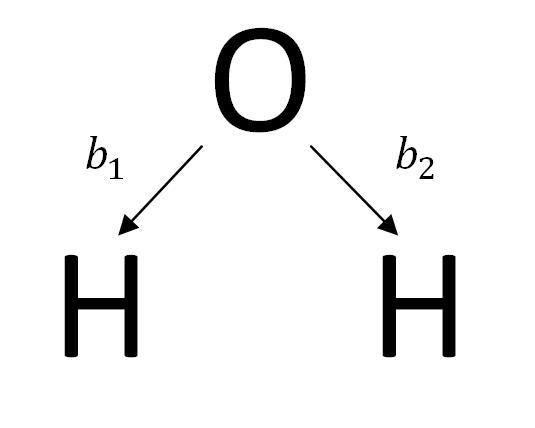Figure $$\PageIndex{2}$$: Labeled vectors of H2O.

Next, the basis vector, v, is transformed by Tj, the jth symmetry operation of the molecule’s point group. As the vector of the basis set is transformed, record the vector that takes its place. Water is a member of the point group C2V. The Symmetry elements of the C2V point group are E, C2, σv, σv’.

The ith SALC function, ϕiis shown below using the vector v=b1.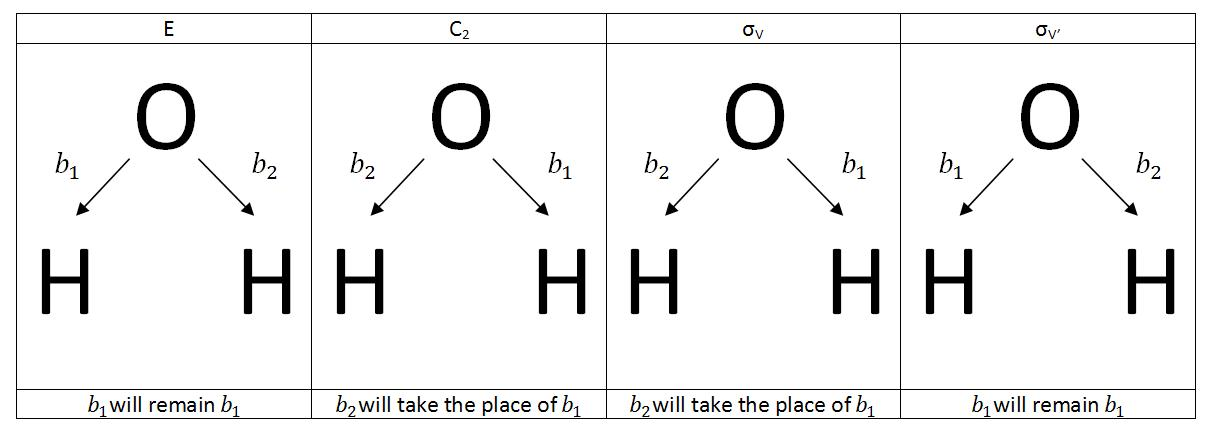Figure $$\PageIndex{3}$$: The transformations of the basis vectors of H2O.

Once the transformations have been determined, the SALC can be constructed by taking the sum of the products of each character of a representation within the point group and the corresponding transformation. The SALCs functions are the collective transformations of the basis sets represented by ϕiwhere Xi(j) is the character of the ith irreducible representation and the jth symmetry operation.

$\phi_{i}=\displaystyle\sum_{j}X_{i}(j)T_{j}\nu \label{7}$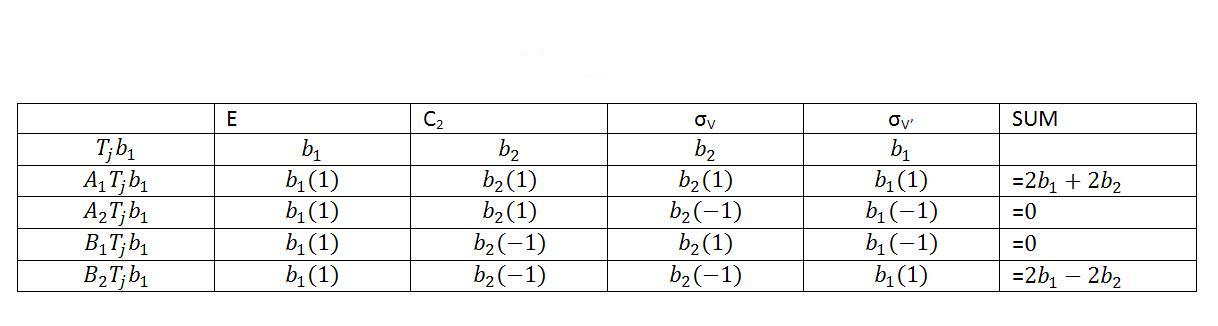Table $$\PageIndex{3}$$: Projection Operator method for C2v

The final step in constructing the SALCs of water is to normalize expressions.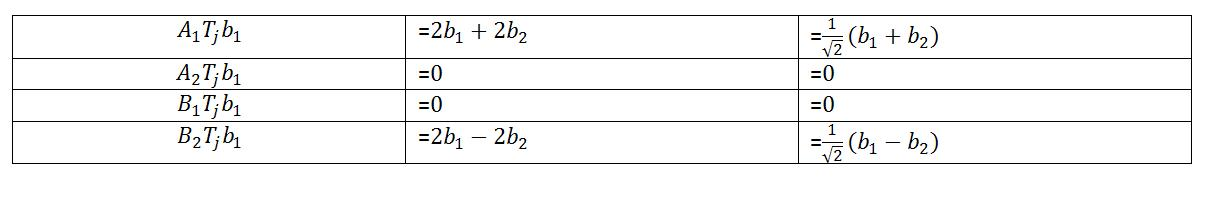Table $$\PageIndex{4}$$: Normalized SALCs of H2O

There are two SALCs for the water molecule, ϕ1(A1) and ϕ1(B2). This demonstrates that water has two stretching modes, one is a totally symmetric stretch with the symmetry, A1, and the other is an antisymmetric stretch with the symmetry B2.Figure $$\PageIndex{4}$$: The stretching modes of H2O resulting from Method 2.

## Interpreting SALCs

Both methods of construction result in the same SALCs. Only irreducible representations corresponding to the symmetries of the stretching modes of the molecule will produce a SALC that is non-zero. Method 1 only utilized the known symmetries of the vibrational modes. All irreducible representations of the point group were used, but the representations that were not vibrational modes resulted in SALCs equal to zero.

Therefore, with the SALCs of a molecule given, all the symmetries of the stretching modes are identified. This allows for a clearer understanding of the spectroscopy of the molecule. Even though vibrational modes can be observed in both infrared and Raman spectroscopy, the SALCs of a molecule cannot identify the magnitude or frequency of the peak in the spectra. The normalized SALCs can, however, help to determine the relative magnitude of the stretching vectors. The magnitude can be determined by the equation below.

$a \cdot b= |a||b|cos \theta \label{8}$

The resulting A1 and B1 symmetries for the above water example are each active in both Raman and IR spectroscopies, according to the C2v character table. If the vibrational mode allows for a change in the dipole moment, the mode can be observed through infrared spectroscopy. If the vibrational mode allows for a change in the polarization of the molecule, the mode can be observed through Raman spectroscopy. Both stretching and bending modes are seen in the spectra, however only stretching modes are expressed in the SALCs.

##### Example $$\PageIndex{2}$$: Difluorobenzene

The SALCs of a molecule can also provide insight to the geometry of a molecule. For example, SALCs can aid in determining the differences between para-difluorobenzene and ortho-difluorobenzene. The SALCs for these two molecules are given below.Figure $$\PageIndex{5}$$: para-difluorobenzene

$\phi (A_{g})= \dfrac{1}{2} (b_{1} + b_{2} + b_{3} +b_{4})$

$\phi (B_{1g})= \dfrac{1}{2} (b_{1} - b_{2} + b_{3} -b_{4})$

$\phi (B_{2u})= \dfrac{1}{2} (b_{1} - b_{2} - b_{3} +b_{4})$

$\phi (B_{3u})= \dfrac{1}{2} (b_{1} + b_{2} - b_{3} -b_{4})$Figure $$\PageIndex{6}$$: ortho-difluorobenzene

$\phi _{1} (A_{1})= \dfrac{1}{\sqrt{2}} (b_{1} + b_{2} + b_{3} +b_{4})$

$\phi _{2} (A_{1})= \dfrac{1}{\sqrt{2}} (b_{1} - b_{2} + b_{3} -b_{4})$

$\phi _{1} (B_{1})= \dfrac{1}{\sqrt{2}} (b_{1} - b_{2} - b_{3} +b_{4})$

$\phi _{2} (B_{1})= \dfrac{1}{\sqrt{2}} (b_{1} + b_{2} - b_{3} -b_{4})$

From the SALCs, it is seen that para-difluorobenzene has four stretching modes and ortho-difluorobenzene has only two. Therefore, it is no surprise that the vibrational spectroscopy of the para-difluorobenzene shows more peaks than the ortho-difluorobenzene.Figure $$\PageIndex{7}$$: FT-IR Raman Spectrum of para-difluorobenzene4Figure $$\PageIndex{8}$$: FT-IR Raman Spectrum of ortho-difluorobenzene5

## Applications

The SALCs of a molecule can be used to understand the stretching modes and binding schemes of a molecule. More information can also be interpreted when applying the projection operator used in SALCs on the atomic orbitals of the molecule. This results in the determination of the linear combination of atomic orbitals (LCAO), which gives information on the molecular orbitals of the molecule. The molecular orbitals (MO) of a molecule are often constructed as LCAOs. Each MO is a solution to the Schrödinger equation and is an eigenfunction of the Hamiltonian operator. The LCAOs can be determined in the same manner as the SALCs of a molecule, with the use of a projection operator. The difference is that the basis set is no longer stretching vectors, but instead the atomic orbitals of the molecule. Hydrogen only has s orbitals, but oxygen has s and p orbitals, where the px, py, and pz all transform differently and therefore must be treated differently.

Once the LCAOs of the molecule have been determined, the expressions can be interpreted into images of the orbitals bonding. If two orbitals are of the same sign in the expression, the electrons in the orbitals are in phase with each other and are bonding. If two orbitals are of the opposite sign in the expression, the electrons in the orbitals are out of phase with each other and are antibonding. The image below shows the atomic orbitals' phases (or signs) as red or blue lobes.Figure $$\PageIndex{9}$$: Atomic Orbitals of H2O.

Any separation between two antibonding atomic orbitals is a planar node. As the number of nodes increases, so does the level of antibonding. This allows for the LCAO to place the molecular orbitals in order of increasing energy, which can be used in constructing the molecular orbital (MO) diagram of the molecule. The irreducible representation used to construct the LCAO is used to describe the MOs. The LCAOs for water are shown below with red dotted lines showing the nodes. Notice the nodes for the px orbitals are in a different plane than the s orbitals of the hydrogens, so these are degenerate and nonbonding.Figure $$\PageIndex{10}$$: Molecular Orbital diagram for H2O in order of increasing energy.

Information from the LCAO of water can also be used to analyze and anticipate the adsorption of water onto various surfaces. Evarestov and Bandura used this technique to identify the water adsorption on Y-doped BaZrO3 and TiO2 (Rutile) respectively.2,3

##### 2

Applying a combination of Methods 1 and 2, the SALCs for CBr2H2 can be determined. The point group of this molecule is C2v, making it similar to the determination of SALCs for water.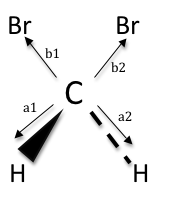Figure $$\PageIndex{11}$$: CBr2H2, point group C2v.

However, the central carbon contains more than one type of attached atom; therefore, the stretching analysis must be performed in pieces. First, the C-H stretches are examined, followed by the C-Br stretches:

Table 5: Irreducible Representations for C-H and C-Br stretches in CBr2H2.
C2V E C2 σV σV’ Irreducible Representation
ΓC-H 2 0 0 2 ΓC-H = A1 + B2
ΓC-Br 2 0 2 0 ΓC-Br = A1 + B1

Applying the projection operator method to C-Br and C-H stretches individually, the SALCs are obtained in the same fashion as before.

Table 6: SALCs for CBr2H2.
ΓC-Br E C2 σV σV’ SUM
A1Tj(b1) b1 b2 b1 b2 2(b1 + b2)
B1Tj(b1) b1 -b2 b1 -b2 2(b1 - b2)
Table 7: SALCs for CBr2H2.
ΓC-H E C2 σV σV’ SUM
A1Tj(a1) a1 a2 a2 a1 2(a1 + a2)
B2Tj(a1) a1 -a2 -a2 a1 2(a1 - a2)

The results are normalized and the following SALCs are obtained for the C2v molecule CBr2H2:

$\phi CBr(A_{1})=\frac{1}{\sqrt{2}}(b_{1}+b_{2})$

$\phi CBr(B_{1}) =\frac{1}{\sqrt{2}} (b_{1}-b_{2})$

$\phi CH(A_{1}) =\frac{1}{\sqrt{2}} (a_{1}+a_{2})$

$\phi CH(B_{2}) =\frac{1}{\sqrt{2}} (a_{1}-a_{2})$

##### 4

To obtain the SALCs for PtCl4, the same general method is applied.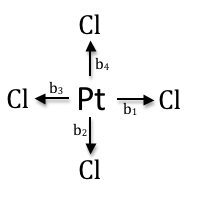Figure $$\PageIndex{12}$$: PtCl4, point group D4h.

However, even though the point group of the molecule is D4h, the cyclic subgroup C4 may be used (this is a more simplified character table used for spherically symmetrical molecules). Some manipulation is required in order to use this cyclic subgroup and will be discussed. Below is the C4 cyclic character table.

Table $$\PageIndex{8}$$: C4 cyclic character table.
C4 E C41 C42 C43
A 1 1 1 1
B 1 -1 1 -1
E1 E2 1 1 i -i -1 -1 -i i

Notice, there are two rows for E, each singly degenerate. To solve for the characters of E, one must take the sum and difference of the two rows. Then, a reduction can be applied to obtain the easiest possible characters by dividing each row by a common factor (removing the common factor is not necessary, but it does simplify the problem as well as remove any imaginary terms):

Sum = [ (1+1) (i-i) (-1-1) (-i+i) ] = (2 0 -2 0) ÷ 2 = E1 (1 0 -1 0)

Difference = [ (1-1) (i+i) (-1+1) (-i-i) ] = (0 2i 0 -2i) ÷ 2i = E2 (0 1 0 -1)

Using the above cyclic group, and the newly obtained characters for E, the projection operator can be applied using Method 2 for the construction of SALCs.

Table $$\PageIndex{9}$$: SALCs for PtCl4 using Method 2.
C4 E C41 C42 C43 SUM
ATj(b1) b1 b2 b3 b4 b1 + b2 + b3 + b4
BTj(b1) b1 -b2 b3 -b4 b1 - b2 + b3 - b4
E1Tj(b1) b1 0 -b3 0 b1 - b3
E2Tj(b1) 0 b2 0 -b4 b2 - b4

Normalizing the sum as mentioned in Method 1, the following SALCs are obtained for the D4h molecule PtCl4:

$\phi (A) =\frac{1}{2} (b_{1}+b_{2}+b_{3}+b_{4})$

$\phi (B)=\frac{1}{2} (b_{1}-b_{2}+b_{3}-b_{4})$

$\phi (E^{1})=\frac{1}{\sqrt{2}} (b_{1}-b_{3})$

$\phi (E^{2})=\frac{1}{\sqrt{2}} (b_{2}-b_{4})$

##### 3

Applying a combination of Methods 1 and 2, the SALCs for the C-H stretches of PF2H3 can be determined. The point group of this molecule is Cs. The central carbon contains more than one type of attached hydrogen; therefore, the stretching analysis must be performed in pieces. First, the C-HA stretches are examined, followed by the C-HB stretches: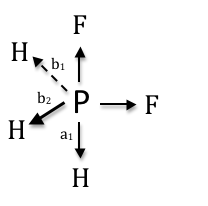Figure $$\PageIndex{13}$$: PF2H3, point group Cs.
Table $$\PageIndex{10}$$: Irreducible Representations for C-Ha and C-Hb stretches in PF2H3.
Cs E σh Irreducible Representation
ΓC-Ha 2 0 ΓC-Ha = A’ + A”
ΓC-Hb 1 1 ΓC-Hb = A’

Applying the projection operator method to C-HA and C-HB stretches individually, the SALCs are obtained in the same fashion as before.

Table $$\PageIndex{11}$$: SALCs for PF2H3 using Method 2.
ΓC-HB E σh SUM
A’ Tj(b1) b1 b2 b1 + b2
A” Tj(b1) b1 -b2 b1 - b2
A’ Tj(a1) a1 a1 a1 + a1
Table $$\PageIndex{12}$$: SALCs for PF2H3 using Method 2.
ΓC-HA E C2 σV σV’ SUM
A1Tj(a1) a1 a2 a2 a1 2(a1+ a2)
B2Tj(a1) a1 -a2 -a2 a1 2(a1- a2)

The results are normalized and the following SALCs are obtained for the Cs molecule PF2H3:

$\phi_{1} A'=\frac{1}{\sqrt{2}} (b_{1} +b_{2} )+a_{1} = \frac{1}{sqrt{3}} (b_{1} +b_{2} +a_{1})$

$\phi_{2} A'=\frac{1}{\sqrt{2}} (b_{1} +b_{2} )-a_{1} = \frac{1}{sqrt{3}} (b_{1} +b_{2} -a_{1})$

$\phi A''=\frac{1}{\sqrt{2}} (b_{1} -b_{2} )$

## Problems

1. Construct the SALCs for C-H stretches of ortho-difluorobenzene.
2. Construct the SALCs for ammonia.
3. Draw the nodes for the MOs of BeH2 (determined by used of LCAOs) and rank the MOs in order of increasing energy.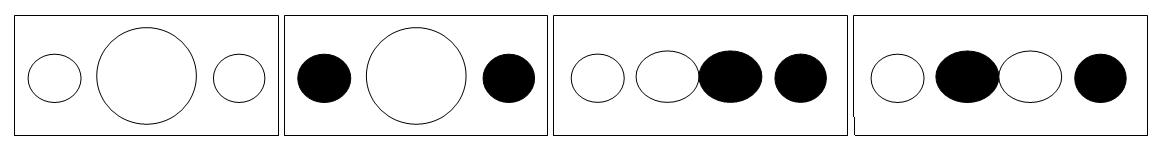## References

1. Willock, David J. Molecular Symmetry. Chichester, U.K: Wiley, 2009.
2. R.A. Evarestov, A.V. Bandura, LCAO calculation of water adsorption on (001) surface of Y-doped BaZrO3, Solid State Ionics, In Press, Corrected Proof, Available online 2 October 2010, ISSN 0167-2738, DOI: 10.1016/j.ssi.2010.09.012.
(www.sciencedirect.com/science...8ab4e49bd4429e)
3. A. V. Bandura,, D. G. Sykes,, V. Shapovalov,, T. N. Troung,, J. D. Kubicki,, and, R. A. Evarestov. Adsorption of Water on the TiO2 (Rutile) (110) Surface:  A Comparison of Periodic and Embedded Cluster Calculations. The Journal of Physical Chemistry B 2004 108 (23), 7844-7853.
4. "1,4-Difluorobenzene" Sigma Aldrich. www.sigmaaldrich.com/catalog/...0&QS=ON&F=SPEC. 14 March, 2011.
5. "1,2-Difluorobenzene" Sigma Aldrich. www.sigmaaldrich.com/catalog/...AND_KEY&F=SPEC. 14 March, 2011.